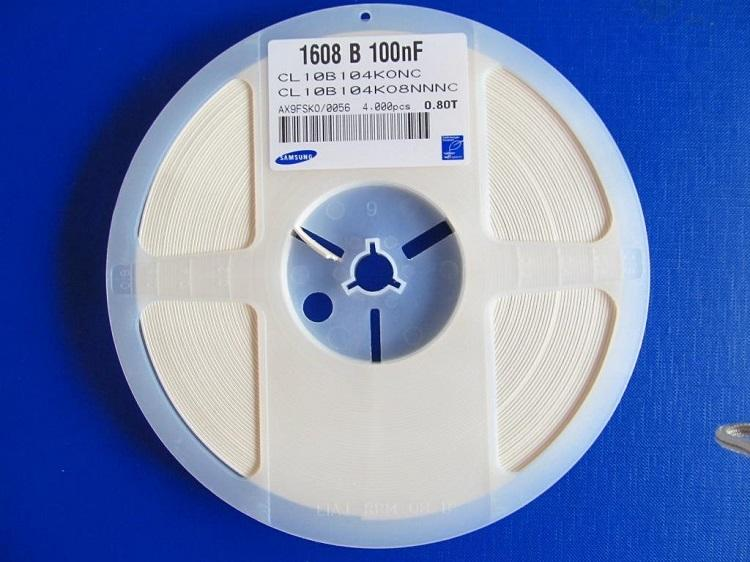﻿ 三星贴片电容的额定电压列表

# 三星贴片电容的额定电压列表- R = 4V
- O = 16V
- B = 50V
- E = 250V
- I = 1000V
- Q = 6.3V
- A = 25V
- C = 100V
- G = 500V
- J = 2000V
- P = 10V
- L = 35V
- D = 200V
- H = 630V
- K = 3000V